﻿ Stress Intensity Factor Solutions | MechaniCalc

Contents

## Center Through Crack in Plate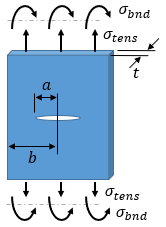Stress intensity: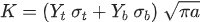Geometry factor, tension: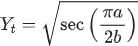Geometry factor, bending: Yb = Yt / 2

Definitions:

 σt tensile stress σb bending stress a crack half-length b plate half-width

References:

1. AFRL-VA-WP-TR-2003-3002, "USAF Damage Tolerant Design Handbook: Guidelines for the Analysis and Design of Damage Tolerant Aircraft Structures," 2002
2. API 579-1 / ASME FFS-1, "Fitness-For-Service," The American Petroleum Institute and The American Society of Mechanical Engineers, 2007

## Single Edge Through Crack in Plate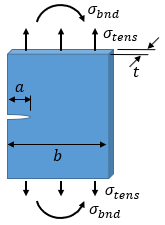Stress intensity:Geometry factor, tension: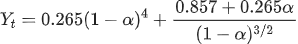Geometry factor, bending: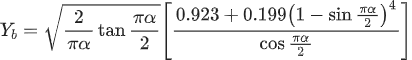Dimension ratio: α = a / b

Definitions:

 σt tensile stress σb bending stress a crack length b plate width

References:

## Elliptical Surface Crack in Plate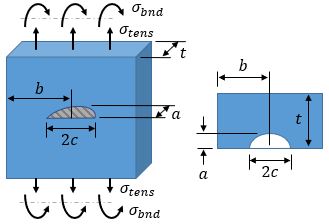Stress intensity:Geometry factor, tension: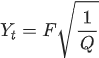Geometry factor, bending: Yb = H·Yt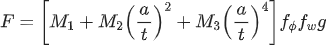$$H = H1 + (H2 − H1)(sin ϕ)p$$ ϕ = 0° at c-tip,  ϕ = 90° at a-tip (full depth of crack)
a ≤ c a > c
---
---

Definitions:

 σt tensile stress σb bending stress a crack depth c crack half-length b plate half-width t plate thickness

References:

## Corner Surface Crack in Plate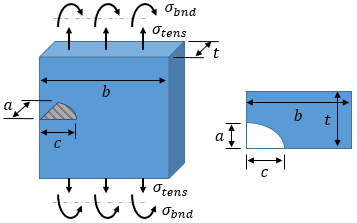Stress intensity:Geometry factor, tension:Geometry factor, bending: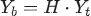$$H = H1 + (H2 − H1)(sin ϕ)p$$ ϕ = 0° at c-tip,  ϕ = 90° at a-tip
a ≤ c a > c
---
---

Definitions:

 σt tensile stress σb bending stress a crack depth c crack length b plate width

References:

## Thumbnail Crack in Solid Cylinder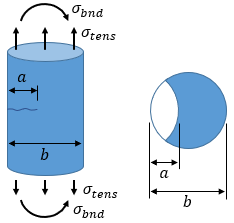Stress intensity:Geometry factor, tension: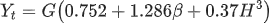Geometry factor, bending: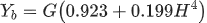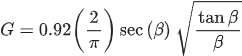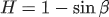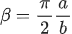Definitions:

 σt tensile stress σb bending stress a crack depth b cylinder diameter

References:

1. AFRL-VA-WP-TR-2003-3002, "USAF Damage Tolerant Design Handbook: Guidelines for the Analysis and Design of Damage Tolerant Aircraft Structures," 2002